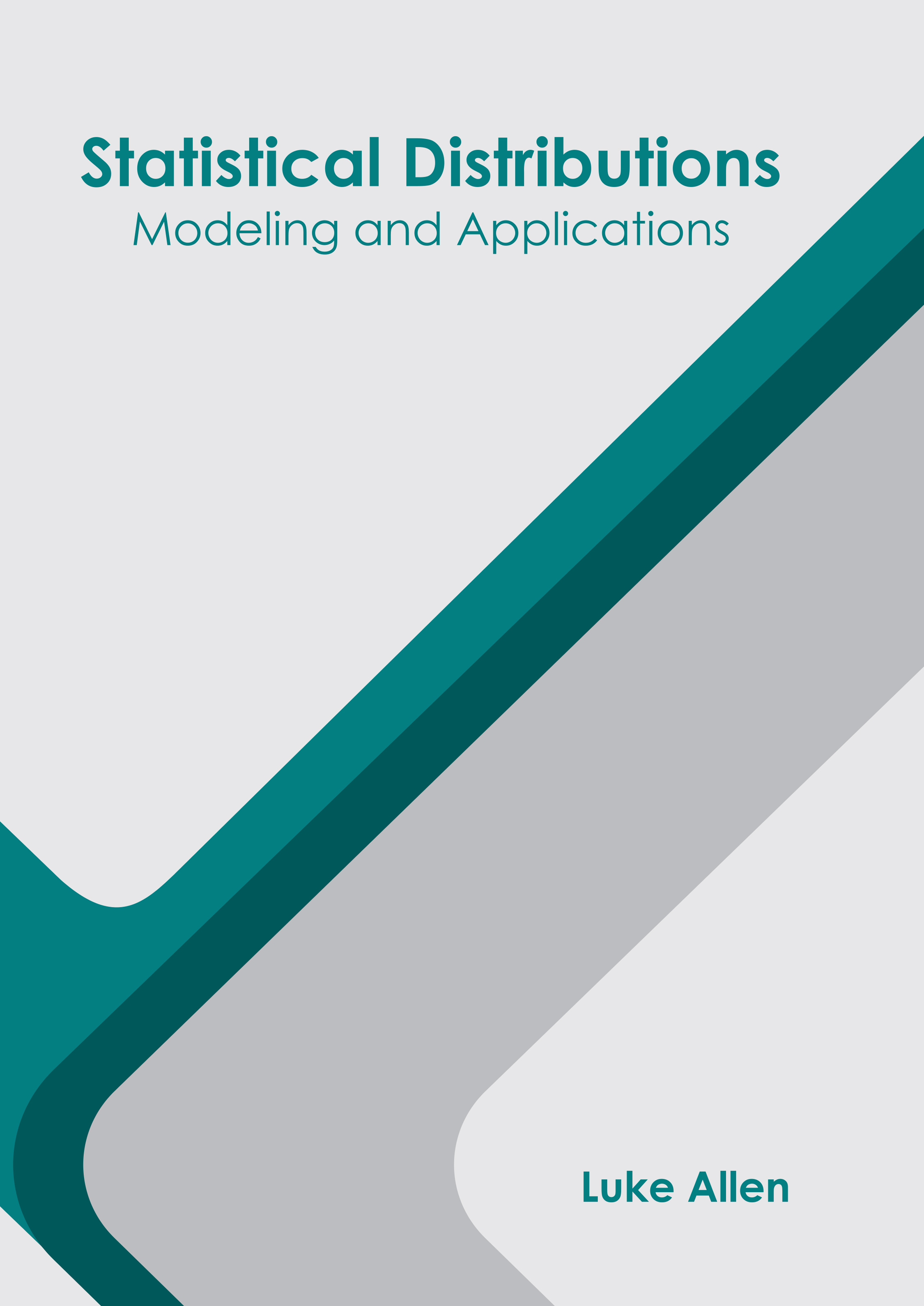BROWSE BY

#### Statistical Distributions: Modeling and Applications9781647253554

Author

Luke Allen

##### Publisher

NY Research Press

English

2021

##### Category

Mathematics - Mathematics

\$ 154.95

##### Description

A statistical distribution is a parameterized mathematical function that gives the probabilities of different outcomes for a random variable. There are discrete and continuous distributions depending on the random value it models. Some of the most prominent statistical distributions are Bernoulli distribution, binomial distribution, geometric distribution, uniform distribution, normal distribution, Poisson distribution, and exponential distribution. This group of statistical distributions has plentiful applications to studies in statistics and probability and it also helps in solving real problems. Understanding statistical distributions plays a very important role for data scientists in order to know the data more rigorously, conduct better data analysis, and choose the more suitable model. This book provides significant information of this discipline to help develop a good understanding of statistical distributions and related fields. The topics included herein are of utmost significance and bound to provide incredible insights to readers. This book is a vital tool for all researching and studying in this field.